# User:IssaRice/Conjunction of subset statements versus Cartesian product subset statement

Jump to: navigation, search

This is an exercise from Tao's Analysis I (exercise 3.5.6) and from Munkres's Topology (exercise 1.1.2(k)–(l)). It is an exercise that makes apparent some of the subtleties of the basic logic used in math.

The exercise is to consider the sets$A,B,C,D$ and the two statements:

1.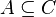$A \subseteq C$ and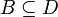$B \subseteq D$; and
2.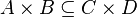$A\times B \subseteq C\times D$;

and to ask whether one implies the other (or whether the two statements are equivalent).

## Relationship between the statements

In general, (1) implies (2). In other words, if$A \subseteq C$ and$B \subseteq D$, then$A\times B \subseteq C\times D$.

If$A$ and$B$ are nonempty sets, then (1) and (2) are logically equivalent. In other words,$A \subseteq C$ and$B \subseteq D$ if and only if$A\times B \subseteq C\times D$.

If$A$ and$B$ are both empty, then (1) and (2) are logically equivalent; in fact, they are both true.

## Each statement written out in first-order logic

1.$\forall x (x \in A \implies x \in C) \wedge \forall y (y \in B \implies y \in D)$
2.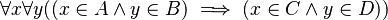$\forall x \forall y ((x \in A \wedge y \in B) \implies (x \in C \wedge y \in D))$

## Erroneous general proof

Here is an erroneous general proof that (2) implies (1).

Exercise. Identify the error in the proof.

Expand to see solution:

blah

## Counterexample

Here is a counterexample that shows that in general, (2) does not imply (1). Let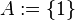$A := \{1\}$,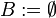$B := \emptyset$,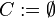$C := \emptyset$, and$D := \{1\}$. Then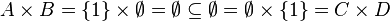$A\times B = \{1\}\times \emptyset =\emptyset \subseteq \emptyset = \emptyset \times \{1\} = C \times D$

but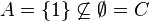$A = \{1\} \not\subseteq \emptyset = C$

Thus (2) is true but (1) is false.